# 2 5 Quadratic Functions Maxima and Minima The

• Slides: 14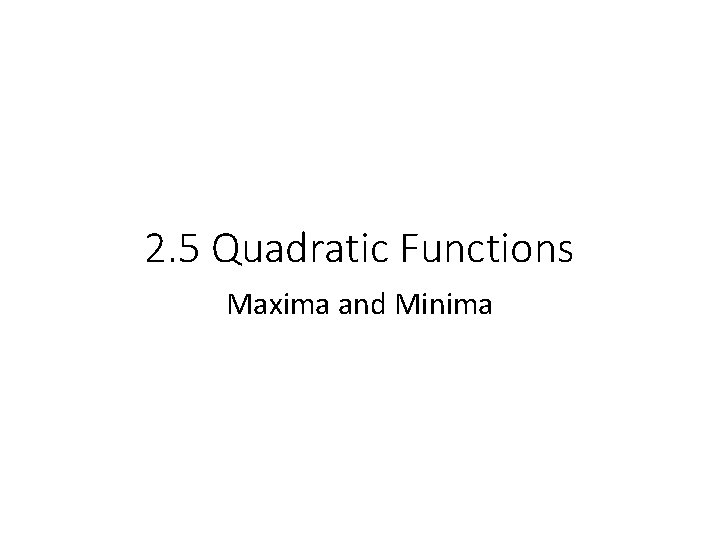2. 5 Quadratic Functions Maxima and Minima• The maximum or minimum value of a function is the largest or smallest value of the function on an interval. • Relative (local)max/min: the greatest or least value that the function can attain on some interval. • If a relative max/min is the greatest or least value of the function over its entire domain, then it is the absolute max or min.• Relative maximum – when the y-coordinate is not the greatest value of the function, although it represents a maximum for a small interval. • Relative minimum – the least y-value on some interval of the domain. • Absolute Max – the greatest value that a function assumes over its domain • Absolute Min – the least value of a function • Extrema are values of the function – they are the y-coordinates of each max and min point.Find the relative and absolute max or min.Parabola’s • Standard Form: y = ax 2 + bx + c • C is the y-intercept • To convert from standard to vertex form you must CTS! • Vertex Form: y = a(x – h)2 + k • Vertex is at (h, k)Write in vertex form and graph: y = 3 x 2 – 6 x + 10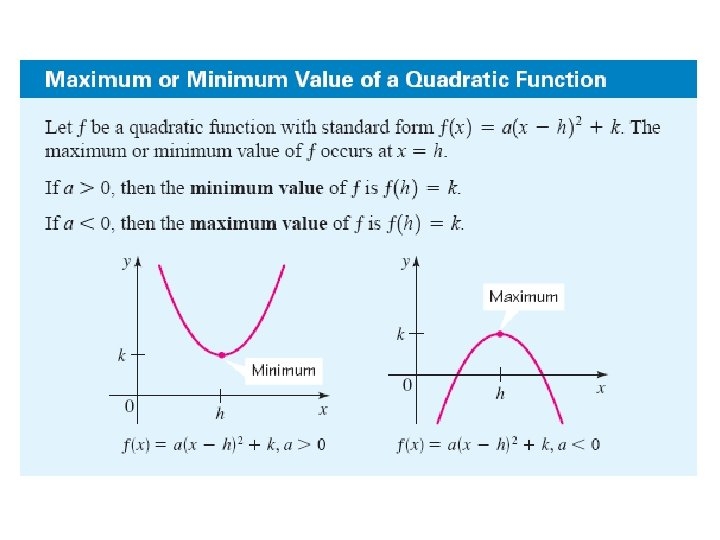Write in vertex form, graph and find the max or min: y = 2 x 2 – 20 x + 53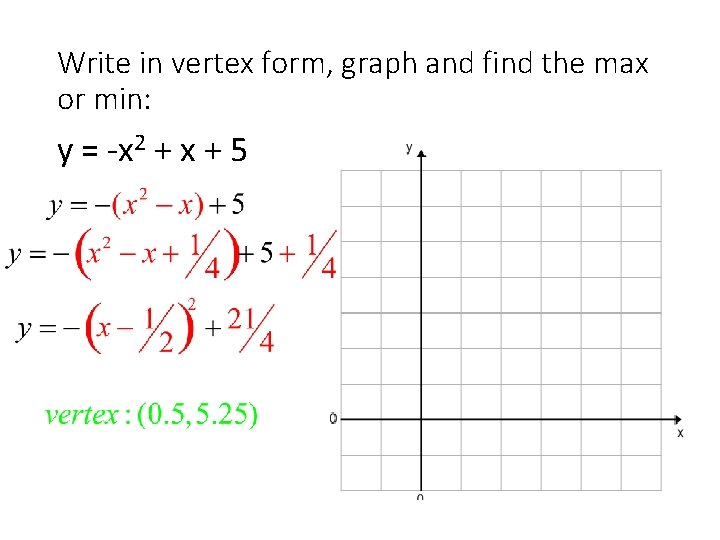Write in vertex form, graph and find the max or min: y = -x 2 + x + 5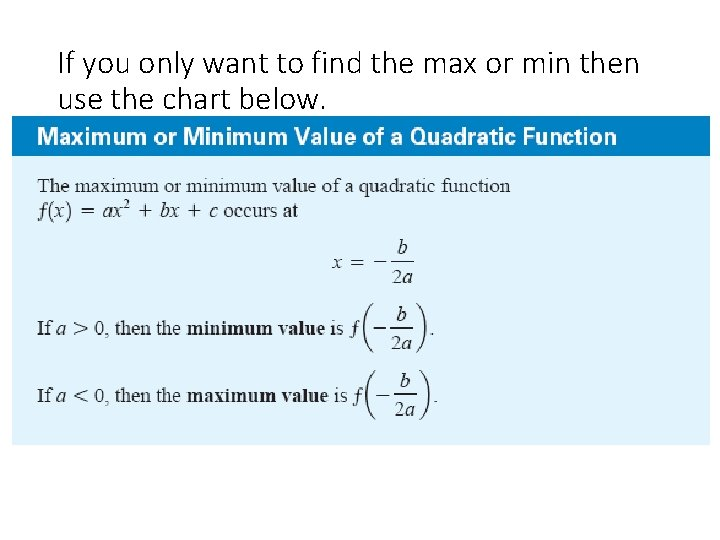If you only want to find the max or min then use the chart below.Find the max or min: • f(x) = x 2 + 8 x f(x) = -4 x 2 + 8 x - 2• Most cars get their best gas mileage when traveling at a relatively modest speed. The gas mileage M for a certain new car is given by , where the speed is between 15 and 70 miles per hour. What is the car’s best gas mileage and at what speed is it attained? Max or min?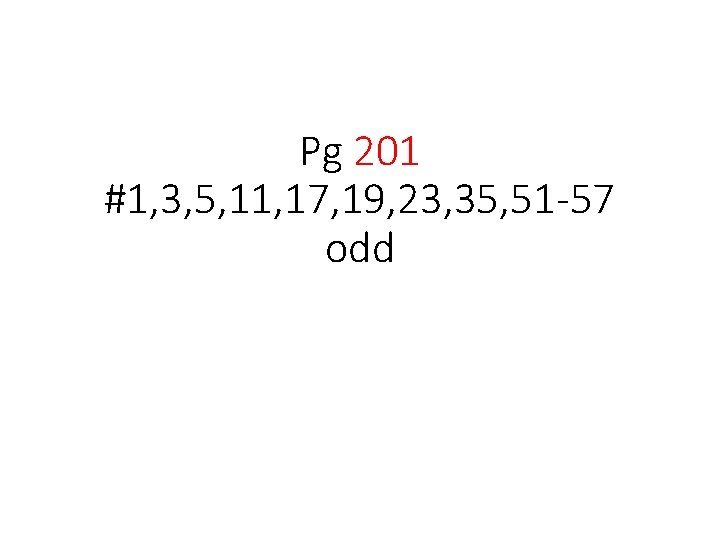Pg 201 #1, 3, 5, 11, 17, 19, 23, 35, 51 -57 odd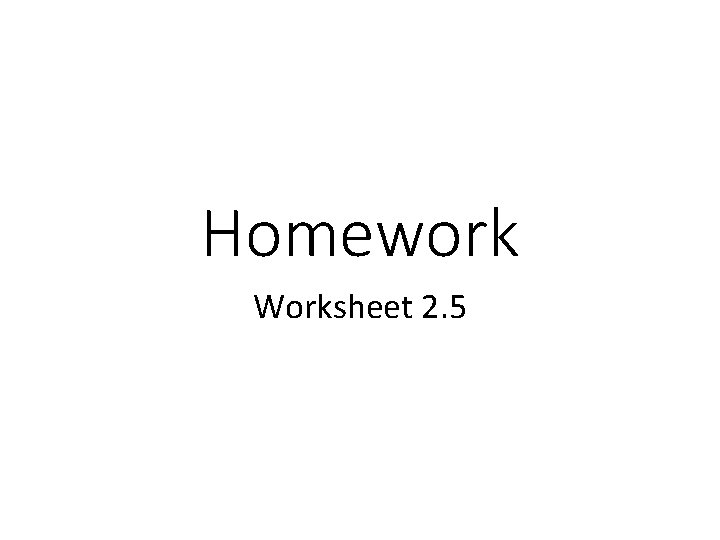Homework Worksheet 2. 5